# EZ-RTE

The energy density for the three-dimensional radiative transport equation (RTE) with anisotropic scattering is calculated without any approximation. See Report by Mihai V. Avram. The analytical solution is based on Machida, JOSAA, 2014. The density of light is obtained as a function of the distance from an isotropic source.

 Chi Zhang and Manabu Machida (Hamamatsu University School of Medicine) Hamamatsu, Japan, December 2022

Go to JavaScript

 Theory The specific intensity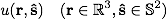of light in a three-dimensional infinite medium with a point source at the origin obeys the following RTE.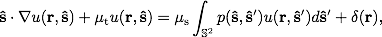where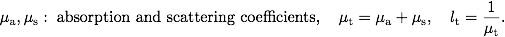Here we assume linear scattering and the scattering phase function is given by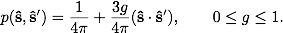The energy density U is obtained as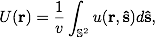where v is the speed of light in the medium. Since U is spherically symmetric, we measure U along the z-axis. We have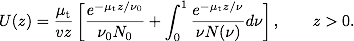See the report for furhter details.

 Input μa: the absorption coefficient ( > 0 ). μs: the scattering coefficient ( > 0 ). g: the scattering asymmetry parameter ( 0 ≤ g ≤ 1 ). * The computation might be difficult for μa ≫ μs since in this case U becomes very small.

 Output In the left panel, we plot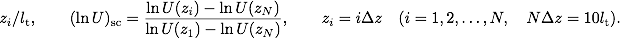In the right panel,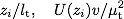are shown.

### Change log

• [December 23, 2022] The JavaScript version was released.
• The initial Java version can be found here (July 15, 2013).